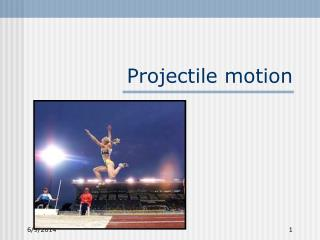# Projectile motion - PowerPoint PPT PresentationDownload PresentationProjectile motion

Projectile motionDownload Presentation## Projectile motion

- - - - - - - - - - - - - - - - - - - - - - - - - - - E N D - - - - - - - - - - - - - - - - - - - - - - - - - - -
##### Presentation Transcript

1. Projectile motion

2. Factors influencing projectile trajectory • Projection angle • Projection speed • Height of projection

3. Definitions • Trajectory: flight path • Angle of projection: direction at which a body is projected with respect to the horizontal

4. Definitions • Projection speed: magnitude of projection velocity • Relative projection height: the difference between projection height and landing height

5. Projection angle • + air resistance governs trajectory shape • Three general shapes: Oblique Horizontal Vertical

6. Projection angle • What would the trajectory path look like with: • A projection angle of 10° • A projection angle of 80° • How does projection angle relate to basketball shooting?

7. Projection speed • Determines length/size of trajectory • Ex: vertical projection • If angle is oblique: speed will determine height AND horizontal length of trajectory • Range: horizontal displacement at landing

8. Projection speed • How does take-off speed affect vertical jump performance? • Are there activities/skills where take-off speed are important?

9. Relative projection height • ‘the difference between projection height and landing height’ • Example: discus throw • Other examples in sports?

10. Projection height • > height = longer flight time & > horizontal displacement • Diving example

11. Optimum projection conditions • Speed of projection • Speed vs height??? • Most varied factor = angle • ? Optimum angle if height = 0 • ? What happens to optimum angle as projection height increases

12. Jumping activities • Which is more important to the long jumper, triple jumper, high jumper, and pole vaulter? (hint: all take off from height of 0) • Take-off angle (projection angle) • Take-off velocity (projection velocity)

13. Analyzing projectile motion • Remember velocity is a vector • Magnitude and direction • Initial velocity has speed and direction (angle) • Horizontal and vertical components

14. Analyzing projectile motion • Horizontal component of velocity is constant • Vertical is constantly changing • Horizontal acceleration = 0 • Vertical acceleration = -9.81m/s2

15. Equations • Laws of constant acceleration • Using s, v, a, & t • Vf = vi + at • S = vit + (1/2)at2 • Vf2 = vi2 + 2as

16. Equations - horizontal • Remove a from equations • Vf = vi • S = vit • Vf2 = vi2

17. Equations - vertical • a = -9.81m/s2 • Vf = at • S = (1/2)at2 • Vf2 = 2as

18. S = 1/2at2 t = 2s/a t = 2(100m) -9.81m/s2 t = 4.5 s Vertical Sx = vt Sx = 30m/s*4.5s Sx = 135 m Solution

19. Equation • To find the apex • 0 = vi2 + 2as To find the flight time: • 0 = vi + at

20. A field goal is being kicked from a distance of 29m from the goal posts. If the horizontal component of the initial velocity = 18m/s and the flight time was 2 s, is the kick long enough? Solution? Problem

21. Tomorrow • Kinemtaic problems • Angular kinematics - gait